## Introduction¶

This notebook aims at getting a good insight in the data for the PorteSeguro competition. Besides that, it gives some tips and tricks to prepare your data for modeling. The notebook consists of the following main sections:

In :
import pandas as pd
import numpy as np
import matplotlib.pyplot as plt
import seaborn as sns
from sklearn.preprocessing import Imputer
from sklearn.preprocessing import PolynomialFeatures
from sklearn.preprocessing import StandardScaler
from sklearn.feature_selection import VarianceThreshold
from sklearn.feature_selection import SelectFromModel
from sklearn.utils import shuffle
from sklearn.ensemble import RandomForestClassifier

pd.set_option('display.max_columns', 100)


In :
train = pd.read_csv('../input/train.csv')


## Data at first sight¶

Here is an excerpt of the the data description for the competition:

• Features that belong to similar groupings are tagged as such in the feature names (e.g., ind, reg, car, calc).
• Feature names include the postfix bin to indicate binary features and cat to indicate categorical features.
• Features without these designations are either continuous or ordinal.
• Values of -1 indicate that the feature was missing from the observation.
• The target columns signifies whether or not a claim was filed for that policy holder.

Ok, that's important information to get us started. Let's have a quick look at the first and last rows to confirm all of this.

In :
train.head()

Out:
id target ps_ind_01 ps_ind_02_cat ps_ind_03 ps_ind_04_cat ps_ind_05_cat ps_ind_06_bin ps_ind_07_bin ps_ind_08_bin ps_ind_09_bin ps_ind_10_bin ps_ind_11_bin ps_ind_12_bin ps_ind_13_bin ps_ind_14 ps_ind_15 ps_ind_16_bin ps_ind_17_bin ps_ind_18_bin ps_reg_01 ps_reg_02 ps_reg_03 ps_car_01_cat ps_car_02_cat ps_car_03_cat ps_car_04_cat ps_car_05_cat ps_car_06_cat ps_car_07_cat ps_car_08_cat ps_car_09_cat ps_car_10_cat ps_car_11_cat ps_car_11 ps_car_12 ps_car_13 ps_car_14 ps_car_15 ps_calc_01 ps_calc_02 ps_calc_03 ps_calc_04 ps_calc_05 ps_calc_06 ps_calc_07 ps_calc_08 ps_calc_09 ps_calc_10 ps_calc_11 ps_calc_12 ps_calc_13 ps_calc_14 ps_calc_15_bin ps_calc_16_bin ps_calc_17_bin ps_calc_18_bin ps_calc_19_bin ps_calc_20_bin
0 7 0 2 2 5 1 0 0 1 0 0 0 0 0 0 0 11 0 1 0 0.7 0.2 0.718070 10 1 -1 0 1 4 1 0 0 1 12 2 0.400000 0.883679 0.370810 3.605551 0.6 0.5 0.2 3 1 10 1 10 1 5 9 1 5 8 0 1 1 0 0 1
1 9 0 1 1 7 0 0 0 0 1 0 0 0 0 0 0 3 0 0 1 0.8 0.4 0.766078 11 1 -1 0 -1 11 1 1 2 1 19 3 0.316228 0.618817 0.388716 2.449490 0.3 0.1 0.3 2 1 9 5 8 1 7 3 1 1 9 0 1 1 0 1 0
2 13 0 5 4 9 1 0 0 0 1 0 0 0 0 0 0 12 1 0 0 0.0 0.0 -1.000000 7 1 -1 0 -1 14 1 1 2 1 60 1 0.316228 0.641586 0.347275 3.316625 0.5 0.7 0.1 2 2 9 1 8 2 7 4 2 7 7 0 1 1 0 1 0
3 16 0 0 1 2 0 0 1 0 0 0 0 0 0 0 0 8 1 0 0 0.9 0.2 0.580948 7 1 0 0 1 11 1 1 3 1 104 1 0.374166 0.542949 0.294958 2.000000 0.6 0.9 0.1 2 4 7 1 8 4 2 2 2 4 9 0 0 0 0 0 0
4 17 0 0 2 0 1 0 1 0 0 0 0 0 0 0 0 9 1 0 0 0.7 0.6 0.840759 11 1 -1 0 -1 14 1 1 2 1 82 3 0.316070 0.565832 0.365103 2.000000 0.4 0.6 0.0 2 2 6 3 10 2 12 3 1 1 3 0 0 0 1 1 0
In :
train.tail()

Out:
id target ps_ind_01 ps_ind_02_cat ps_ind_03 ps_ind_04_cat ps_ind_05_cat ps_ind_06_bin ps_ind_07_bin ps_ind_08_bin ps_ind_09_bin ps_ind_10_bin ps_ind_11_bin ps_ind_12_bin ps_ind_13_bin ps_ind_14 ps_ind_15 ps_ind_16_bin ps_ind_17_bin ps_ind_18_bin ps_reg_01 ps_reg_02 ps_reg_03 ps_car_01_cat ps_car_02_cat ps_car_03_cat ps_car_04_cat ps_car_05_cat ps_car_06_cat ps_car_07_cat ps_car_08_cat ps_car_09_cat ps_car_10_cat ps_car_11_cat ps_car_11 ps_car_12 ps_car_13 ps_car_14 ps_car_15 ps_calc_01 ps_calc_02 ps_calc_03 ps_calc_04 ps_calc_05 ps_calc_06 ps_calc_07 ps_calc_08 ps_calc_09 ps_calc_10 ps_calc_11 ps_calc_12 ps_calc_13 ps_calc_14 ps_calc_15_bin ps_calc_16_bin ps_calc_17_bin ps_calc_18_bin ps_calc_19_bin ps_calc_20_bin
595207 1488013 0 3 1 10 0 0 0 0 0 1 0 0 0 0 0 13 1 0 0 0.5 0.3 0.692820 10 1 -1 0 1 1 1 1 0 1 31 3 0.374166 0.684631 0.385487 2.645751 0.4 0.5 0.3 3 0 9 0 9 1 12 4 1 9 6 0 1 1 0 1 1
595208 1488016 0 5 1 3 0 0 0 0 0 1 0 0 0 0 0 6 1 0 0 0.9 0.7 1.382027 9 1 -1 0 -1 15 0 0 2 1 63 2 0.387298 0.972145 -1.000000 3.605551 0.2 0.2 0.0 2 4 8 6 8 2 12 4 1 3 8 1 0 1 0 1 1
595209 1488017 0 1 1 10 0 0 1 0 0 0 0 0 0 0 0 12 1 0 0 0.9 0.2 0.659071 7 1 -1 0 -1 1 1 1 2 1 31 3 0.397492 0.596373 0.398748 1.732051 0.4 0.0 0.3 3 2 7 4 8 0 10 3 2 2 6 0 0 1 0 0 0
595210 1488021 0 5 2 3 1 0 0 0 1 0 0 0 0 0 0 12 1 0 0 0.9 0.4 0.698212 11 1 -1 0 -1 11 1 1 2 1 101 3 0.374166 0.764434 0.384968 3.162278 0.0 0.7 0.0 4 0 9 4 9 2 11 4 1 4 2 0 1 1 1 0 0
595211 1488027 0 0 1 8 0 0 1 0 0 0 0 0 0 0 0 7 1 0 0 0.1 0.2 -1.000000 7 0 -1 0 -1 0 1 0 2 1 34 2 0.400000 0.932649 0.378021 3.741657 0.4 0.0 0.5 2 3 10 4 10 2 5 4 4 3 8 0 1 0 0 0 0

We indeed see the following

• binary variables
• categorical variables of which the category values are integers
• other variables with integer or float values
• variables with -1 representing missing values
• the target variable and an ID variable

Let's look at the number of rows and columns in the train data.

In :
train.shape

Out:
(595212, 59)

We have 59 variables and 595.212 rows. Let's see if we have the same number of variables in the test data.
Let's see if there are duplicate rows in the training data.

In :
train.drop_duplicates()
train.shape

Out:
(595212, 59)

No duplicate rows, so that's fine.

In :
test.shape

Out:
(892816, 58)

We are missing one variable in the test set, but this is the target variable. So that's fine.
Let's now invesigate how many variables of each type we have.

So later on we can create dummy variables for the 14 categorical variables. The bin variables are already binary and do not need dummification.

In :
train.info()

<class 'pandas.core.frame.DataFrame'>
RangeIndex: 595212 entries, 0 to 595211
Data columns (total 59 columns):
id                595212 non-null int64
target            595212 non-null int64
ps_ind_01         595212 non-null int64
ps_ind_02_cat     595212 non-null int64
ps_ind_03         595212 non-null int64
ps_ind_04_cat     595212 non-null int64
ps_ind_05_cat     595212 non-null int64
ps_ind_06_bin     595212 non-null int64
ps_ind_07_bin     595212 non-null int64
ps_ind_08_bin     595212 non-null int64
ps_ind_09_bin     595212 non-null int64
ps_ind_10_bin     595212 non-null int64
ps_ind_11_bin     595212 non-null int64
ps_ind_12_bin     595212 non-null int64
ps_ind_13_bin     595212 non-null int64
ps_ind_14         595212 non-null int64
ps_ind_15         595212 non-null int64
ps_ind_16_bin     595212 non-null int64
ps_ind_17_bin     595212 non-null int64
ps_ind_18_bin     595212 non-null int64
ps_reg_01         595212 non-null float64
ps_reg_02         595212 non-null float64
ps_reg_03         595212 non-null float64
ps_car_01_cat     595212 non-null int64
ps_car_02_cat     595212 non-null int64
ps_car_03_cat     595212 non-null int64
ps_car_04_cat     595212 non-null int64
ps_car_05_cat     595212 non-null int64
ps_car_06_cat     595212 non-null int64
ps_car_07_cat     595212 non-null int64
ps_car_08_cat     595212 non-null int64
ps_car_09_cat     595212 non-null int64
ps_car_10_cat     595212 non-null int64
ps_car_11_cat     595212 non-null int64
ps_car_11         595212 non-null int64
ps_car_12         595212 non-null float64
ps_car_13         595212 non-null float64
ps_car_14         595212 non-null float64
ps_car_15         595212 non-null float64
ps_calc_01        595212 non-null float64
ps_calc_02        595212 non-null float64
ps_calc_03        595212 non-null float64
ps_calc_04        595212 non-null int64
ps_calc_05        595212 non-null int64
ps_calc_06        595212 non-null int64
ps_calc_07        595212 non-null int64
ps_calc_08        595212 non-null int64
ps_calc_09        595212 non-null int64
ps_calc_10        595212 non-null int64
ps_calc_11        595212 non-null int64
ps_calc_12        595212 non-null int64
ps_calc_13        595212 non-null int64
ps_calc_14        595212 non-null int64
ps_calc_15_bin    595212 non-null int64
ps_calc_16_bin    595212 non-null int64
ps_calc_17_bin    595212 non-null int64
ps_calc_18_bin    595212 non-null int64
ps_calc_19_bin    595212 non-null int64
ps_calc_20_bin    595212 non-null int64
dtypes: float64(10), int64(49)
memory usage: 267.9 MB


Again, with the info() method we see that the data type is integer or float. No null values are present in the data set. That's normal because missing values are replaced by -1. We'll look into that later.

To facilitate the data management, we'll store meta-information about the variables in a DataFrame. This will be helpful when we want to select specific variables for analysis, visualization, modeling, ...

Concretely we will store:

• role: input, ID, target
• level: nominal, interval, ordinal, binary
• keep: True or False
• dtype: int, float, str
In :
data = []
for f in train.columns:
# Defining the role
if f == 'target':
role = 'target'
elif f == 'id':
role = 'id'
else:
role = 'input'

# Defining the level
if 'bin' in f or f == 'target':
level = 'binary'
elif 'cat' in f or f == 'id':
level = 'nominal'
elif train[f].dtype == float:
level = 'interval'
elif train[f].dtype == int:
level = 'ordinal'

# Initialize keep to True for all variables except for id
keep = True
if f == 'id':
keep = False

# Defining the data type
dtype = train[f].dtype

# Creating a Dict that contains all the metadata for the variable
f_dict = {
'varname': f,
'role': role,
'level': level,
'keep': keep,
'dtype': dtype
}
data.append(f_dict)

meta = pd.DataFrame(data, columns=['varname', 'role', 'level', 'keep', 'dtype'])
meta.set_index('varname', inplace=True)

In :
meta

Out:
role level keep dtype
varname
id id nominal False int64
target target binary True int64
ps_ind_01 input ordinal True int64
ps_ind_02_cat input nominal True int64
ps_ind_03 input ordinal True int64
ps_ind_04_cat input nominal True int64
ps_ind_05_cat input nominal True int64
ps_ind_06_bin input binary True int64
ps_ind_07_bin input binary True int64
ps_ind_08_bin input binary True int64
ps_ind_09_bin input binary True int64
ps_ind_10_bin input binary True int64
ps_ind_11_bin input binary True int64
ps_ind_12_bin input binary True int64
ps_ind_13_bin input binary True int64
ps_ind_14 input ordinal True int64
ps_ind_15 input ordinal True int64
ps_ind_16_bin input binary True int64
ps_ind_17_bin input binary True int64
ps_ind_18_bin input binary True int64
ps_reg_01 input interval True float64
ps_reg_02 input interval True float64
ps_reg_03 input interval True float64
ps_car_01_cat input nominal True int64
ps_car_02_cat input nominal True int64
ps_car_03_cat input nominal True int64
ps_car_04_cat input nominal True int64
ps_car_05_cat input nominal True int64
ps_car_06_cat input nominal True int64
ps_car_07_cat input nominal True int64
ps_car_08_cat input nominal True int64
ps_car_09_cat input nominal True int64
ps_car_10_cat input nominal True int64
ps_car_11_cat input nominal True int64
ps_car_11 input ordinal True int64
ps_car_12 input interval True float64
ps_car_13 input interval True float64
ps_car_14 input interval True float64
ps_car_15 input interval True float64
ps_calc_01 input interval True float64
ps_calc_02 input interval True float64
ps_calc_03 input interval True float64
ps_calc_04 input ordinal True int64
ps_calc_05 input ordinal True int64
ps_calc_06 input ordinal True int64
ps_calc_07 input ordinal True int64
ps_calc_08 input ordinal True int64
ps_calc_09 input ordinal True int64
ps_calc_10 input ordinal True int64
ps_calc_11 input ordinal True int64
ps_calc_12 input ordinal True int64
ps_calc_13 input ordinal True int64
ps_calc_14 input ordinal True int64
ps_calc_15_bin input binary True int64
ps_calc_16_bin input binary True int64
ps_calc_17_bin input binary True int64
ps_calc_18_bin input binary True int64
ps_calc_19_bin input binary True int64
ps_calc_20_bin input binary True int64

Example to extract all nominal variables that are not dropped

In :
meta[(meta.level == 'nominal') & (meta.keep)].index

Out:
Index(['ps_ind_02_cat', 'ps_ind_04_cat', 'ps_ind_05_cat', 'ps_car_01_cat',
'ps_car_02_cat', 'ps_car_03_cat', 'ps_car_04_cat', 'ps_car_05_cat',
'ps_car_06_cat', 'ps_car_07_cat', 'ps_car_08_cat', 'ps_car_09_cat',
'ps_car_10_cat', 'ps_car_11_cat'],
dtype='object', name='varname')

Below the number of variables per role and level are displayed.

In :
pd.DataFrame({'count' : meta.groupby(['role', 'level'])['role'].size()}).reset_index()

Out:
role level count
0 id nominal 1
1 input binary 17
2 input interval 10
3 input nominal 14
4 input ordinal 16
5 target binary 1

## Descriptive statistics¶

We can also apply the describe method on the dataframe. However, it doesn't make much sense to calculate the mean, std, ... on categorical variables and the id variable. We'll explore the categorical variables visually later.

Thanks to our meta file we can easily select the variables on which we want to compute the descriptive statistics. To keep things clear, we'll do this per data type.

### Interval variables¶

In :
v = meta[(meta.level == 'interval') & (meta.keep)].index
train[v].describe()

Out:
ps_reg_01 ps_reg_02 ps_reg_03 ps_car_12 ps_car_13 ps_car_14 ps_car_15 ps_calc_01 ps_calc_02 ps_calc_03
count 595212.000000 595212.000000 595212.000000 595212.000000 595212.000000 595212.000000 595212.000000 595212.000000 595212.000000 595212.000000
mean 0.610991 0.439184 0.551102 0.379945 0.813265 0.276256 3.065899 0.449756 0.449589 0.449849
std 0.287643 0.404264 0.793506 0.058327 0.224588 0.357154 0.731366 0.287198 0.286893 0.287153
min 0.000000 0.000000 -1.000000 -1.000000 0.250619 -1.000000 0.000000 0.000000 0.000000 0.000000
25% 0.400000 0.200000 0.525000 0.316228 0.670867 0.333167 2.828427 0.200000 0.200000 0.200000
50% 0.700000 0.300000 0.720677 0.374166 0.765811 0.368782 3.316625 0.500000 0.400000 0.500000
75% 0.900000 0.600000 1.000000 0.400000 0.906190 0.396485 3.605551 0.700000 0.700000 0.700000
max 0.900000 1.800000 4.037945 1.264911 3.720626 0.636396 3.741657 0.900000 0.900000 0.900000

#### reg variables¶

• only ps_reg_03 has missing values
• the range (min to max) differs between the variables. We could apply scaling (e.g. StandardScaler), but it depends on the classifier we will want to use.

#### car variables¶

• ps_car_12 and ps_car_15 have missing values
• again, the range differs and we could apply scaling.

#### calc variables¶

• no missing values
• this seems to be some kind of ratio as the maximum is 0.9
• all three _calc variables have very similar distributions

Overall, we can see that the range of the interval variables is rather small. Perhaps some transformation (e.g. log) is already applied in order to anonymize the data?

### Ordinal variables¶

In :
v = meta[(meta.level == 'ordinal') & (meta.keep)].index
train[v].describe()

Out:
ps_ind_01 ps_ind_03 ps_ind_14 ps_ind_15 ps_car_11 ps_calc_04 ps_calc_05 ps_calc_06 ps_calc_07 ps_calc_08 ps_calc_09 ps_calc_10 ps_calc_11 ps_calc_12 ps_calc_13 ps_calc_14
count 595212.000000 595212.000000 595212.000000 595212.000000 595212.000000 595212.000000 595212.000000 595212.000000 595212.000000 595212.000000 595212.000000 595212.000000 595212.000000 595212.000000 595212.000000 595212.000000
mean 1.900378 4.423318 0.012451 7.299922 2.346072 2.372081 1.885886 7.689445 3.005823 9.225904 2.339034 8.433590 5.441382 1.441918 2.872288 7.539026
std 1.983789 2.699902 0.127545 3.546042 0.832548 1.117219 1.134927 1.334312 1.414564 1.459672 1.246949 2.904597 2.332871 1.202963 1.694887 2.746652
min 0.000000 0.000000 0.000000 0.000000 -1.000000 0.000000 0.000000 0.000000 0.000000 2.000000 0.000000 0.000000 0.000000 0.000000 0.000000 0.000000
25% 0.000000 2.000000 0.000000 5.000000 2.000000 2.000000 1.000000 7.000000 2.000000 8.000000 1.000000 6.000000 4.000000 1.000000 2.000000 6.000000
50% 1.000000 4.000000 0.000000 7.000000 3.000000 2.000000 2.000000 8.000000 3.000000 9.000000 2.000000 8.000000 5.000000 1.000000 3.000000 7.000000
75% 3.000000 6.000000 0.000000 10.000000 3.000000 3.000000 3.000000 9.000000 4.000000 10.000000 3.000000 10.000000 7.000000 2.000000 4.000000 9.000000
max 7.000000 11.000000 4.000000 13.000000 3.000000 5.000000 6.000000 10.000000 9.000000 12.000000 7.000000 25.000000 19.000000 10.000000 13.000000 23.000000
• Only one missing variable: ps_car_11
• We could apply scaling to deal with the different ranges

### Binary variables¶

In :
v = meta[(meta.level == 'binary') & (meta.keep)].index
train[v].describe()

Out:
target ps_ind_06_bin ps_ind_07_bin ps_ind_08_bin ps_ind_09_bin ps_ind_10_bin ps_ind_11_bin ps_ind_12_bin ps_ind_13_bin ps_ind_16_bin ps_ind_17_bin ps_ind_18_bin ps_calc_15_bin ps_calc_16_bin ps_calc_17_bin ps_calc_18_bin ps_calc_19_bin ps_calc_20_bin
count 595212.000000 595212.000000 595212.000000 595212.000000 595212.000000 595212.000000 595212.000000 595212.000000 595212.000000 595212.000000 595212.000000 595212.000000 595212.000000 595212.000000 595212.000000 595212.000000 595212.000000 595212.000000
mean 0.036448 0.393742 0.257033 0.163921 0.185304 0.000373 0.001692 0.009439 0.000948 0.660823 0.121081 0.153446 0.122427 0.627840 0.554182 0.287182 0.349024 0.153318
std 0.187401 0.488579 0.436998 0.370205 0.388544 0.019309 0.041097 0.096693 0.030768 0.473430 0.326222 0.360417 0.327779 0.483381 0.497056 0.452447 0.476662 0.360295
min 0.000000 0.000000 0.000000 0.000000 0.000000 0.000000 0.000000 0.000000 0.000000 0.000000 0.000000 0.000000 0.000000 0.000000 0.000000 0.000000 0.000000 0.000000
25% 0.000000 0.000000 0.000000 0.000000 0.000000 0.000000 0.000000 0.000000 0.000000 0.000000 0.000000 0.000000 0.000000 0.000000 0.000000 0.000000 0.000000 0.000000
50% 0.000000 0.000000 0.000000 0.000000 0.000000 0.000000 0.000000 0.000000 0.000000 1.000000 0.000000 0.000000 0.000000 1.000000 1.000000 0.000000 0.000000 0.000000
75% 0.000000 1.000000 1.000000 0.000000 0.000000 0.000000 0.000000 0.000000 0.000000 1.000000 0.000000 0.000000 0.000000 1.000000 1.000000 1.000000 1.000000 0.000000
max 1.000000 1.000000 1.000000 1.000000 1.000000 1.000000 1.000000 1.000000 1.000000 1.000000 1.000000 1.000000 1.000000 1.000000 1.000000 1.000000 1.000000 1.000000
• A priori in the train data is 3.645%, which is strongly imbalanced.
• From the means we can conclude that for most variables the value is zero in most cases.

## Handling imbalanced classes¶

As we mentioned above the proportion of records with target=1 is far less than target=0. This can lead to a model that has great accuracy but does have any added value in practice. Two possible strategies to deal with this problem are:

• oversampling records with target=1
• undersampling records with target=0

There are many more strategies of course and MachineLearningMastery.com gives a nice overview. As we have a rather large training set, we can go for undersampling.

In :
desired_apriori=0.10

# Get the indices per target value
idx_0 = train[train.target == 0].index
idx_1 = train[train.target == 1].index

# Get original number of records per target value
nb_0 = len(train.loc[idx_0])
nb_1 = len(train.loc[idx_1])

# Calculate the undersampling rate and resulting number of records with target=0
undersampling_rate = ((1-desired_apriori)*nb_1)/(nb_0*desired_apriori)
undersampled_nb_0 = int(undersampling_rate*nb_0)
print('Rate to undersample records with target=0: {}'.format(undersampling_rate))
print('Number of records with target=0 after undersampling: {}'.format(undersampled_nb_0))

# Randomly select records with target=0 to get at the desired a priori
undersampled_idx = shuffle(idx_0, random_state=37, n_samples=undersampled_nb_0)

# Construct list with remaining indices
idx_list = list(undersampled_idx) + list(idx_1)

# Return undersample data frame
train = train.loc[idx_list].reset_index(drop=True)

Rate to undersample records with target=0: 0.34043569687437886
Number of records with target=0 after undersampling: 195246


## Data Quality Checks¶

### Checking missing values¶

Missings are represented as -1

In :
vars_with_missing = []

for f in train.columns:
missings = train[train[f] == -1][f].count()
if missings > 0:
vars_with_missing.append(f)
missings_perc = missings/train.shape

print('Variable {} has {} records ({:.2%}) with missing values'.format(f, missings, missings_perc))

print('In total, there are {} variables with missing values'.format(len(vars_with_missing)))

Variable ps_ind_02_cat has 103 records (0.05%) with missing values
Variable ps_ind_04_cat has 51 records (0.02%) with missing values
Variable ps_ind_05_cat has 2256 records (1.04%) with missing values
Variable ps_reg_03 has 38580 records (17.78%) with missing values
Variable ps_car_01_cat has 62 records (0.03%) with missing values
Variable ps_car_02_cat has 2 records (0.00%) with missing values
Variable ps_car_03_cat has 148367 records (68.39%) with missing values
Variable ps_car_05_cat has 96026 records (44.26%) with missing values
Variable ps_car_07_cat has 4431 records (2.04%) with missing values
Variable ps_car_09_cat has 230 records (0.11%) with missing values
Variable ps_car_11 has 1 records (0.00%) with missing values
Variable ps_car_14 has 15726 records (7.25%) with missing values
In total, there are 12 variables with missing values

• ps_car_03_cat and ps_car_05_cat have a large proportion of records with missing values. Remove these variables.
• For the other categorical variables with missing values, we can leave the missing value -1 as such.
• ps_reg_03 (continuous) has missing values for 18% of all records. Replace by the mean.
• ps_car_11 (ordinal) has only 5 records with misisng values. Replace by the mode.
• ps_car_12 (continuous) has only 1 records with missing value. Replace by the mean.
• ps_car_14 (continuous) has missing values for 7% of all records. Replace by the mean.
In :
# Dropping the variables with too many missing values
vars_to_drop = ['ps_car_03_cat', 'ps_car_05_cat']
train.drop(vars_to_drop, inplace=True, axis=1)
meta.loc[(vars_to_drop),'keep'] = False  # Updating the meta

# Imputing with the mean or mode
mean_imp = Imputer(missing_values=-1, strategy='mean', axis=0)
mode_imp = Imputer(missing_values=-1, strategy='most_frequent', axis=0)
train['ps_reg_03'] = mean_imp.fit_transform(train[['ps_reg_03']]).ravel()
train['ps_car_12'] = mean_imp.fit_transform(train[['ps_car_12']]).ravel()
train['ps_car_14'] = mean_imp.fit_transform(train[['ps_car_14']]).ravel()
train['ps_car_11'] = mode_imp.fit_transform(train[['ps_car_11']]).ravel()


### Checking the cardinality of the categorical variables¶

Cardinality refers to the number of different values in a variable. As we will create dummy variables from the categorical variables later on, we need to check whether there are variables with many distinct values. We should handle these variables differently as they would result in many dummy variables.

In :
v = meta[(meta.level == 'nominal') & (meta.keep)].index

for f in v:
dist_values = train[f].value_counts().shape
print('Variable {} has {} distinct values'.format(f, dist_values))

Variable ps_ind_02_cat has 5 distinct values
Variable ps_ind_04_cat has 3 distinct values
Variable ps_ind_05_cat has 8 distinct values
Variable ps_car_01_cat has 13 distinct values
Variable ps_car_02_cat has 3 distinct values
Variable ps_car_04_cat has 10 distinct values
Variable ps_car_06_cat has 18 distinct values
Variable ps_car_07_cat has 3 distinct values
Variable ps_car_08_cat has 2 distinct values
Variable ps_car_09_cat has 6 distinct values
Variable ps_car_10_cat has 3 distinct values
Variable ps_car_11_cat has 104 distinct values


Only ps_car_11_cat has many distinct values, although it is still reasonable.

EDIT: nickycan made an excellent remark on the fact that my first solution could lead to data leakage. He also pointed me to another kernel made by oliver which deals with that. I therefore replaced this part with the kernel of oliver. All credits go to him. It is so great what you can learn by participating in the Kaggle competitions :)

In :
# Script by https://www.kaggle.com/ogrellier
# Code: https://www.kaggle.com/ogrellier/python-target-encoding-for-categorical-features
return series * (1 + noise_level * np.random.randn(len(series)))

def target_encode(trn_series=None,
tst_series=None,
target=None,
min_samples_leaf=1,
smoothing=1,
noise_level=0):
"""
Smoothing is computed like in the following paper by Daniele Micci-Barreca
https://kaggle2.blob.core.windows.net/forum-message-attachments/225952/7441/high%20cardinality%20categoricals.pdf
trn_series : training categorical feature as a pd.Series
tst_series : test categorical feature as a pd.Series
target : target data as a pd.Series
min_samples_leaf (int) : minimum samples to take category average into account
smoothing (int) : smoothing effect to balance categorical average vs prior
"""
assert len(trn_series) == len(target)
assert trn_series.name == tst_series.name
temp = pd.concat([trn_series, target], axis=1)
# Compute target mean
averages = temp.groupby(by=trn_series.name)[target.name].agg(["mean", "count"])
# Compute smoothing
smoothing = 1 / (1 + np.exp(-(averages["count"] - min_samples_leaf) / smoothing))
# Apply average function to all target data
prior = target.mean()
# The bigger the count the less full_avg is taken into account
averages[target.name] = prior * (1 - smoothing) + averages["mean"] * smoothing
averages.drop(["mean", "count"], axis=1, inplace=True)
# Apply averages to trn and tst series
ft_trn_series = pd.merge(
trn_series.to_frame(trn_series.name),
averages.reset_index().rename(columns={'index': target.name, target.name: 'average'}),
on=trn_series.name,
how='left')['average'].rename(trn_series.name + '_mean').fillna(prior)
# pd.merge does not keep the index so restore it
ft_trn_series.index = trn_series.index
ft_tst_series = pd.merge(
tst_series.to_frame(tst_series.name),
averages.reset_index().rename(columns={'index': target.name, target.name: 'average'}),
on=tst_series.name,
how='left')['average'].rename(trn_series.name + '_mean').fillna(prior)
# pd.merge does not keep the index so restore it
ft_tst_series.index = tst_series.index

In :
train_encoded, test_encoded = target_encode(train["ps_car_11_cat"],
test["ps_car_11_cat"],
target=train.target,
min_samples_leaf=100,
smoothing=10,
noise_level=0.01)

train['ps_car_11_cat_te'] = train_encoded
train.drop('ps_car_11_cat', axis=1, inplace=True)
meta.loc['ps_car_11_cat','keep'] = False  # Updating the meta
test['ps_car_11_cat_te'] = test_encoded
test.drop('ps_car_11_cat', axis=1, inplace=True)


## Exploratory Data Visualization¶

### Categorical variables¶

Let's look into the categorical variables and the proportion of customers with target = 1

In :
v = meta[(meta.level == 'nominal') & (meta.keep)].index

for f in v:
plt.figure()
fig, ax = plt.subplots(figsize=(20,10))
# Calculate the percentage of target=1 per category value
cat_perc = train[[f, 'target']].groupby([f],as_index=False).mean()
cat_perc.sort_values(by='target', ascending=False, inplace=True)
# Bar plot
# Order the bars descending on target mean
sns.barplot(ax=ax, x=f, y='target', data=cat_perc, order=cat_perc[f])
plt.ylabel('% target', fontsize=18)
plt.xlabel(f, fontsize=18)
plt.tick_params(axis='both', which='major', labelsize=18)
plt.show();

<matplotlib.figure.Figure at 0x7ff768a9d668>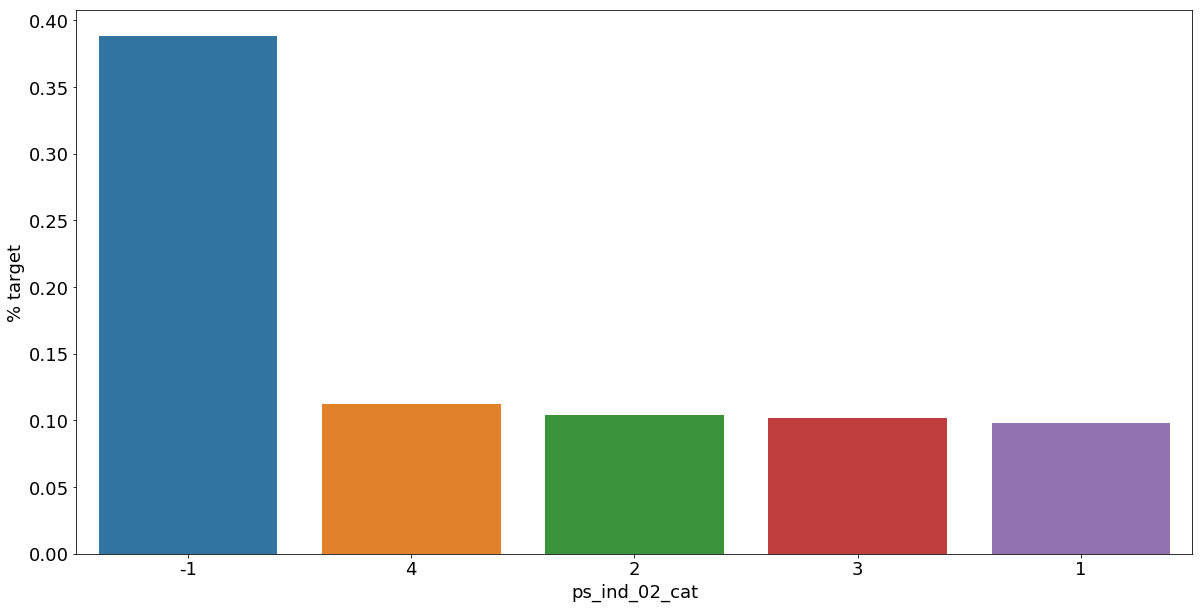<matplotlib.figure.Figure at 0x7ff768a4e080>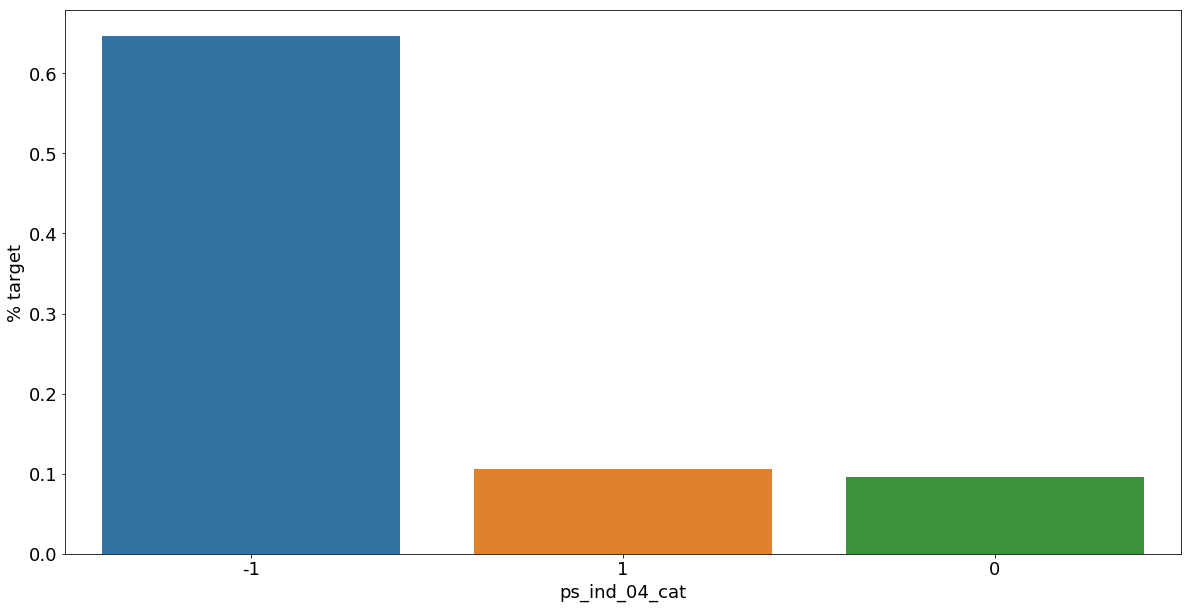<matplotlib.figure.Figure at 0x7ff7688ccac8>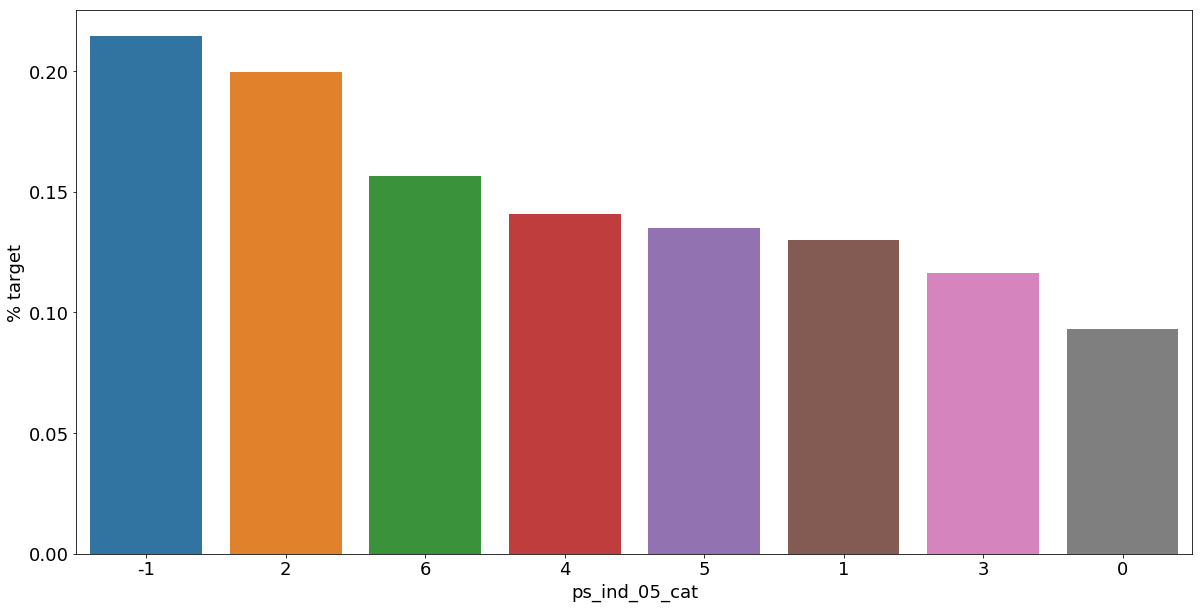<matplotlib.figure.Figure at 0x7ff7689fbba8>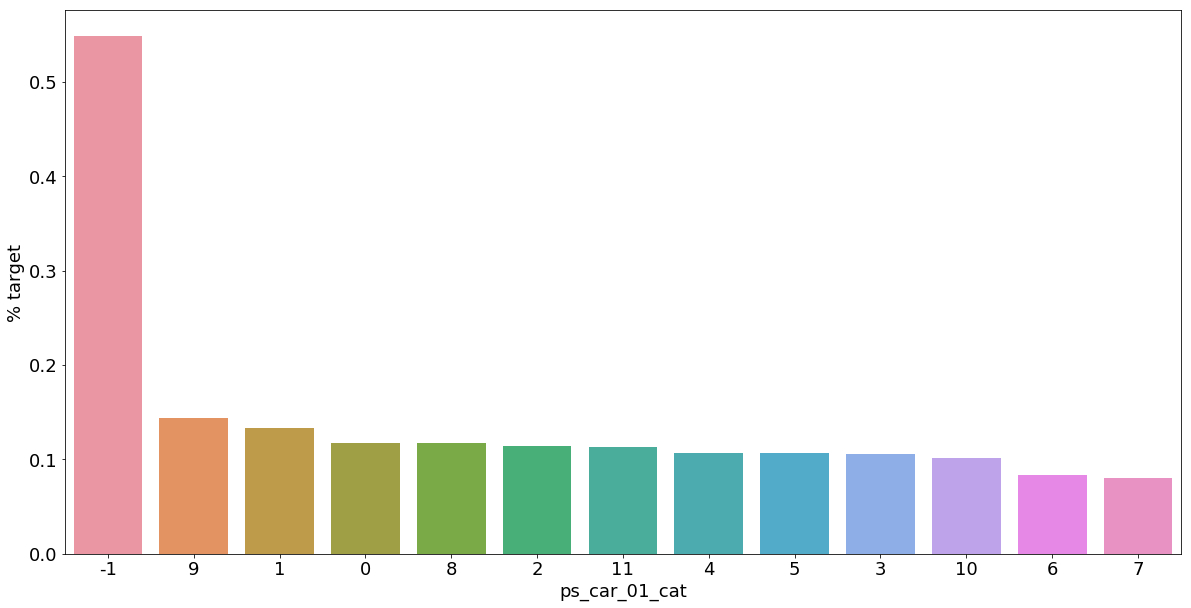<matplotlib.figure.Figure at 0x7ff768a50208>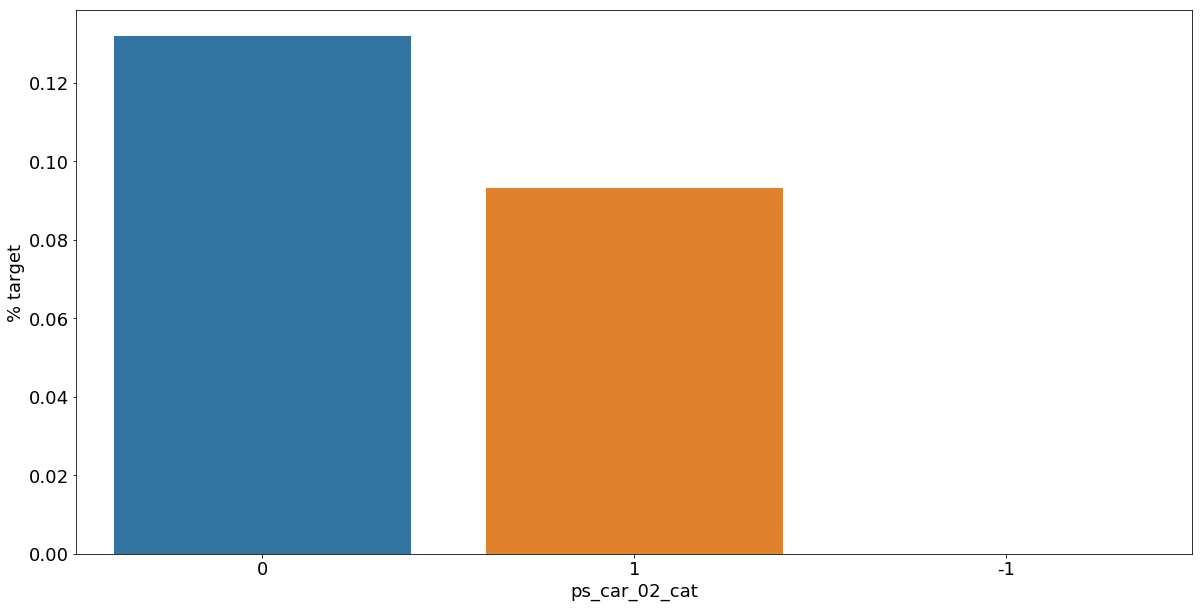<matplotlib.figure.Figure at 0x7ff7686a3eb8>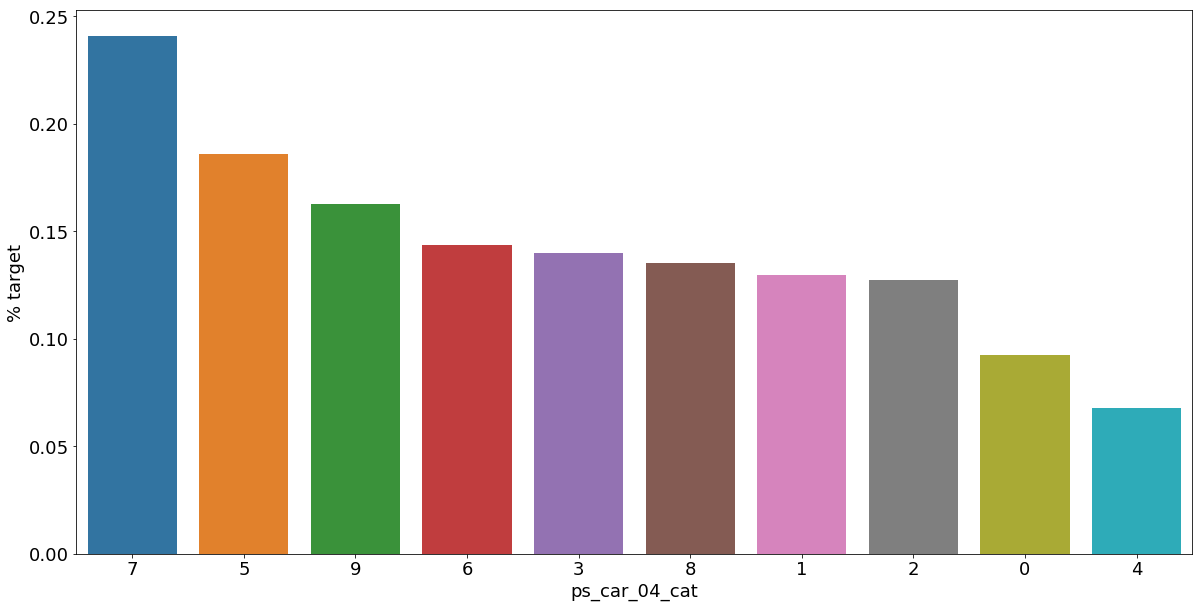<matplotlib.figure.Figure at 0x7ff768735048>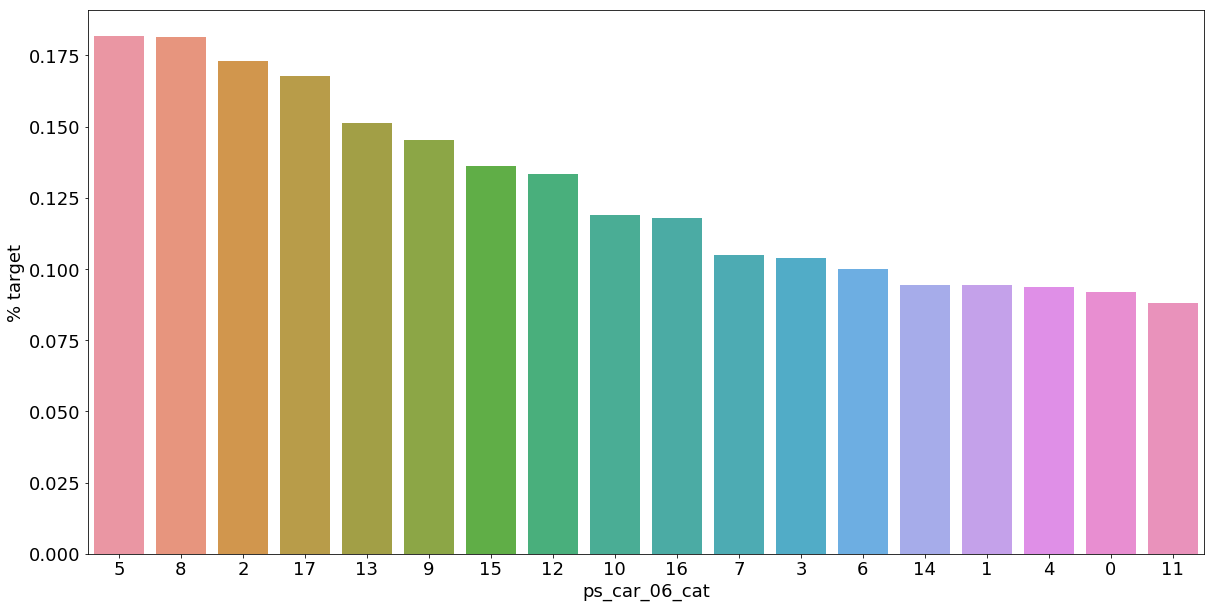<matplotlib.figure.Figure at 0x7ff7685a9080>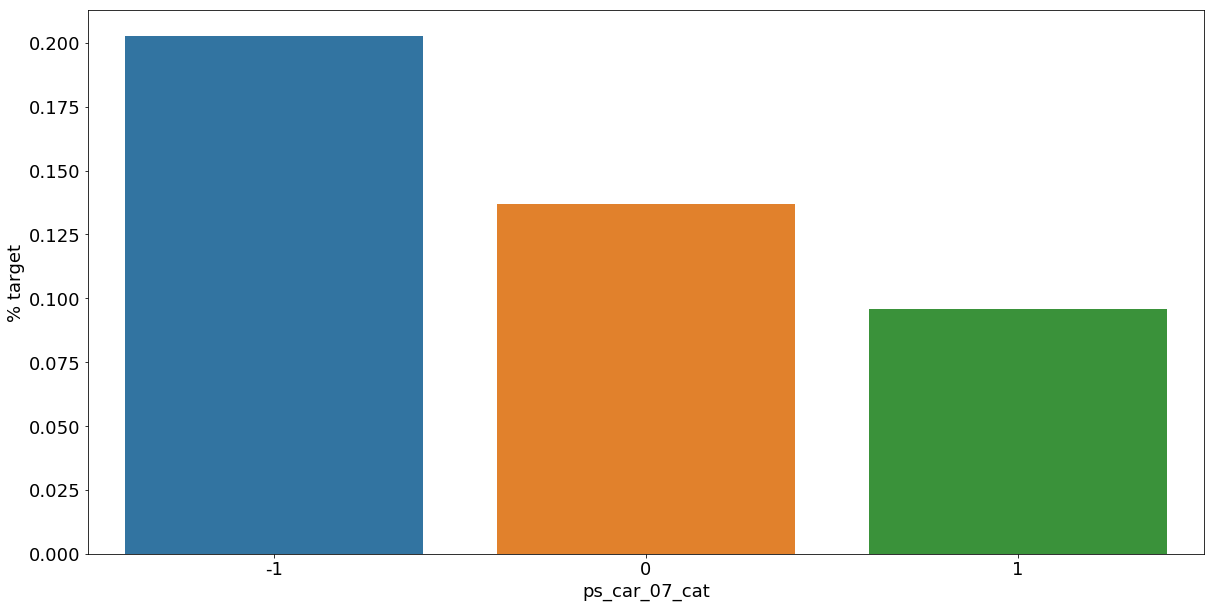<matplotlib.figure.Figure at 0x7ff7683a9be0>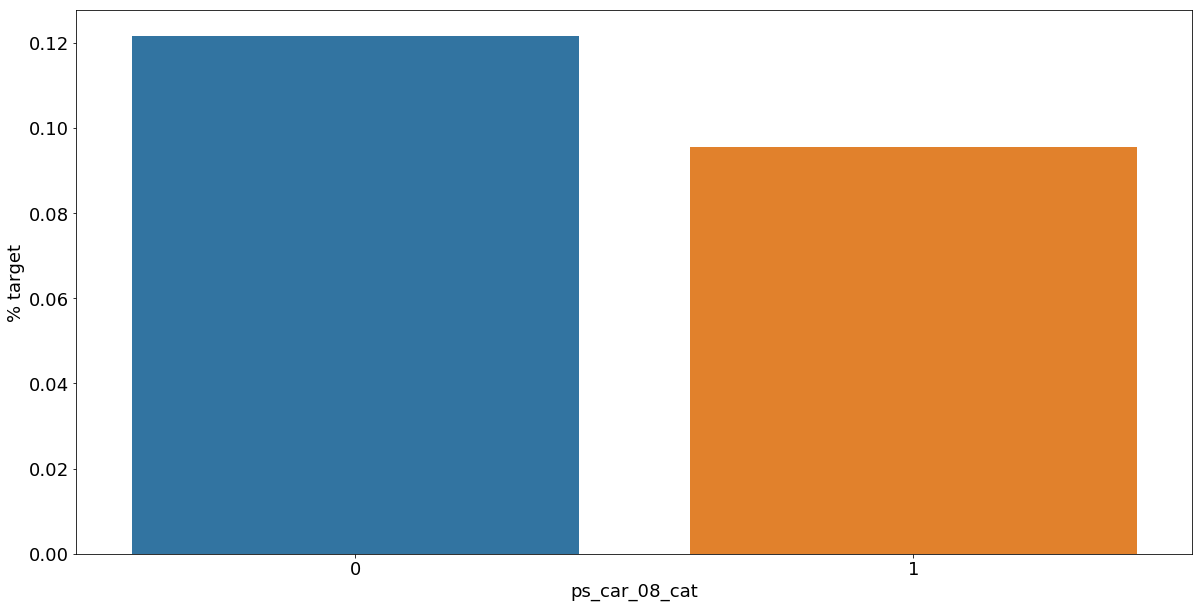<matplotlib.figure.Figure at 0x7ff768438240>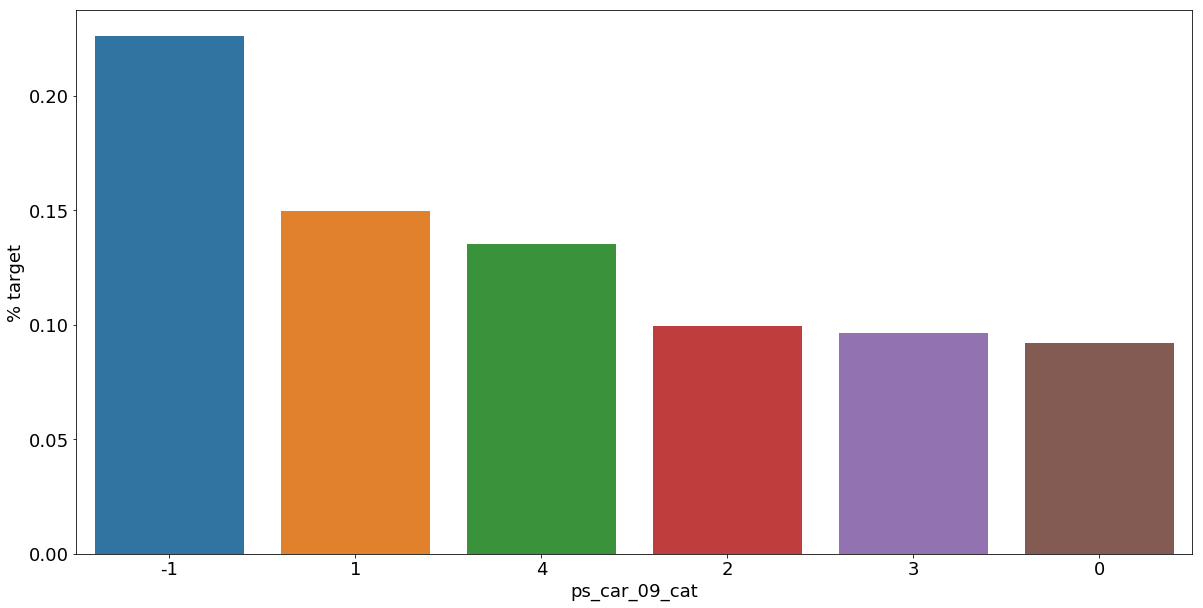<matplotlib.figure.Figure at 0x7ff76847e208>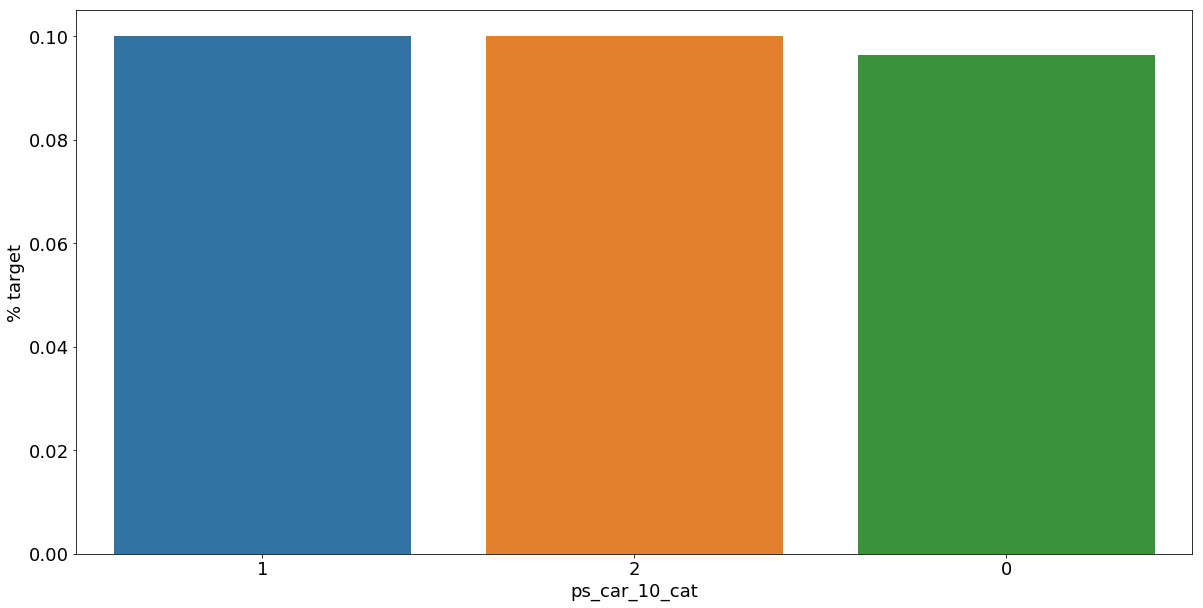As we can see from the variables with missing values, it is a good idea to keep the missing values as a separate category value, instead of replacing them by the mode for instance. The customers with a missing value appear to have a much higher (in some cases much lower) probability to ask for an insurance claim.

### Interval variables¶

Checking the correlations between interval variables. A heatmap is a good way to visualize the correlation between variables. The code below is based on an example by Michael Waskom

In :
def corr_heatmap(v):
correlations = train[v].corr()

# Create color map ranging between two colors
cmap = sns.diverging_palette(220, 10, as_cmap=True)

fig, ax = plt.subplots(figsize=(10,10))
sns.heatmap(correlations, cmap=cmap, vmax=1.0, center=0, fmt='.2f',
square=True, linewidths=.5, annot=True, cbar_kws={"shrink": .75})
plt.show();

v = meta[(meta.level == 'interval') & (meta.keep)].index
corr_heatmap(v)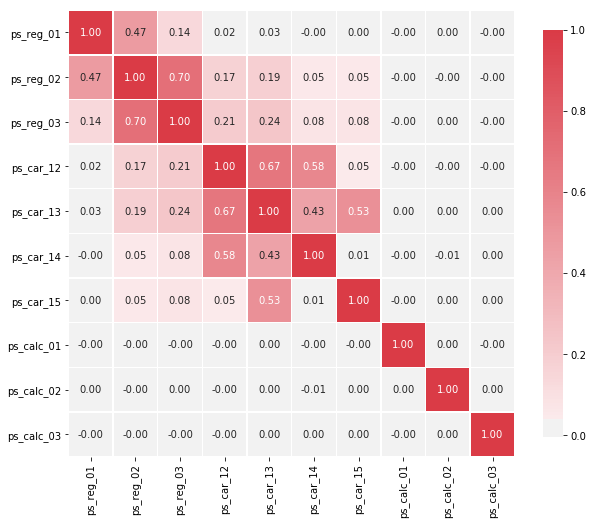There are a strong correlations between the variables:

• ps_reg_02 and ps_reg_03 (0.7)
• ps_car_12 and ps_car13 (0.67)
• ps_car_12 and ps_car14 (0.58)
• ps_car_13 and ps_car15 (0.67)

Seaborn has some handy plots to visualize the (linear) relationship between variables. We could use a pairplot to visualize the relationship between the variables. But because the heatmap already showed the limited number of correlated variables, we'll look at each of the highly correlated variables separately.
NOTE: I take a sample of the train data to speed up the process.

In :
s = train.sample(frac=0.1)


#### ps_reg_02 and ps_reg_03¶

As the regression line shows, there is a linear relationship between these variables. Thanks to the hue parameter we can see that the regression lines for target=0 and target=1 are the same.

In :
sns.lmplot(x='ps_reg_02', y='ps_reg_03', data=s, hue='target', palette='Set1', scatter_kws={'alpha':0.3})
plt.show()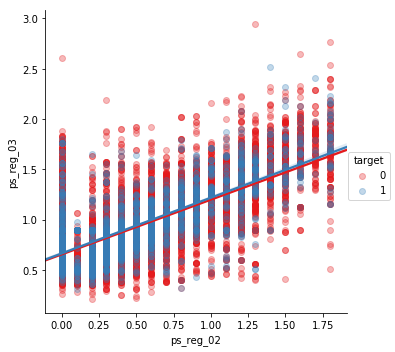#### ps_car_12 and ps_car_13¶

In :
sns.lmplot(x='ps_car_12', y='ps_car_13', data=s, hue='target', palette='Set1', scatter_kws={'alpha':0.3})
plt.show()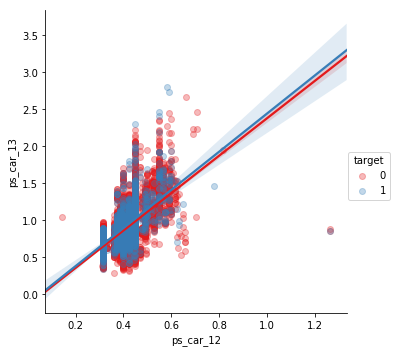#### ps_car_12 and ps_car_14¶

In :
sns.lmplot(x='ps_car_12', y='ps_car_14', data=s, hue='target', palette='Set1', scatter_kws={'alpha':0.3})
plt.show()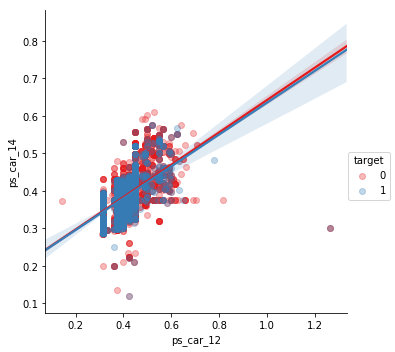#### ps_car_13 and ps_car_15¶

In :
sns.lmplot(x='ps_car_15', y='ps_car_13', data=s, hue='target', palette='Set1', scatter_kws={'alpha':0.3})
plt.show()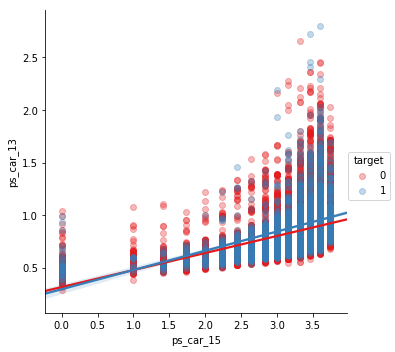Allright, so now what? How can we decide which of the correlated variables to keep? We could perform Principal Component Analysis (PCA) on the variables to reduce the dimensions. In the AllState Claims Severity Competition I made this kernel to do that. But as the number of correlated variables is rather low, we will let the model do the heavy-lifting.

### Checking the correlations between ordinal variables¶

In :
v = meta[(meta.level == 'ordinal') & (meta.keep)].index
corr_heatmap(v)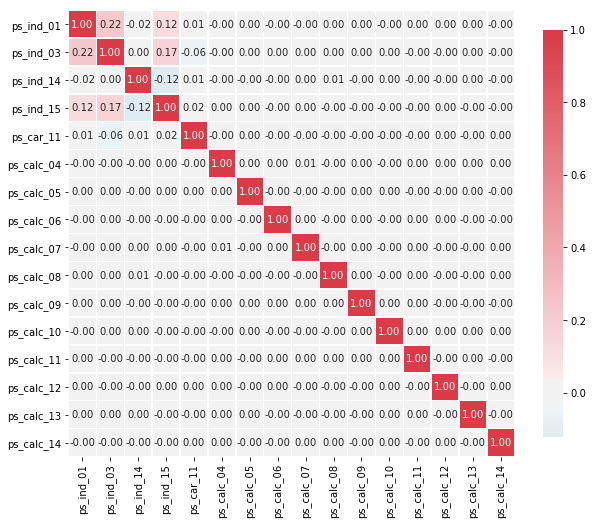For the ordinal variables we do not see many correlations. We could, on the other hand, look at how the distributions are when grouping by the target value.

## Feature engineering¶

### Creating dummy variables¶

The values of the categorical variables do not represent any order or magnitude. For instance, category 2 is not twice the value of category 1. Therefore we can create dummy variables to deal with that. We drop the first dummy variable as this information can be derived from the other dummy variables generated for the categories of the original variable.

In :
v = meta[(meta.level == 'nominal') & (meta.keep)].index
print('Before dummification we have {} variables in train'.format(train.shape))
train = pd.get_dummies(train, columns=v, drop_first=True)
print('After dummification we have {} variables in train'.format(train.shape))

Before dummification we have 57 variables in train
After dummification we have 109 variables in train


So, creating dummy variables adds 52 variables to the training set.

### Creating interaction variables¶

In :
v = meta[(meta.level == 'interval') & (meta.keep)].index
poly = PolynomialFeatures(degree=2, interaction_only=False, include_bias=False)
interactions = pd.DataFrame(data=poly.fit_transform(train[v]), columns=poly.get_feature_names(v))
interactions.drop(v, axis=1, inplace=True)  # Remove the original columns
# Concat the interaction variables to the train data
print('Before creating interactions we have {} variables in train'.format(train.shape))
train = pd.concat([train, interactions], axis=1)
print('After creating interactions we have {} variables in train'.format(train.shape))

Before creating interactions we have 109 variables in train
After creating interactions we have 164 variables in train


This adds extra interaction variables to the train data. Thanks to the get_feature_names method we can assign column names to these new variables.

## Feature selection¶

### Removing features with low or zero variance¶

Personally, I prefer to let the classifier algorithm chose which features to keep. But there is one thing that we can do ourselves. That is removing features with no or a very low variance. Sklearn has a handy method to do that: VarianceThreshold. By default it removes features with zero variance. This will not be applicable for this competition as we saw there are no zero-variance variables in the previous steps. But if we would remove features with less than 1% variance, we would remove 31 variables.

In :
selector = VarianceThreshold(threshold=.01)
selector.fit(train.drop(['id', 'target'], axis=1)) # Fit to train without id and target variables

f = np.vectorize(lambda x : not x) # Function to toggle boolean array elements

v = train.drop(['id', 'target'], axis=1).columns[f(selector.get_support())]
print('{} variables have too low variance.'.format(len(v)))
print('These variables are {}'.format(list(v)))

28 variables have too low variance.
These variables are ['ps_ind_10_bin', 'ps_ind_11_bin', 'ps_ind_12_bin', 'ps_ind_13_bin', 'ps_car_12', 'ps_car_14', 'ps_car_11_cat_te', 'ps_ind_05_cat_2', 'ps_ind_05_cat_5', 'ps_car_01_cat_1', 'ps_car_01_cat_2', 'ps_car_04_cat_3', 'ps_car_04_cat_4', 'ps_car_04_cat_5', 'ps_car_04_cat_6', 'ps_car_04_cat_7', 'ps_car_06_cat_2', 'ps_car_06_cat_5', 'ps_car_06_cat_8', 'ps_car_06_cat_12', 'ps_car_06_cat_16', 'ps_car_06_cat_17', 'ps_car_09_cat_4', 'ps_car_10_cat_1', 'ps_car_10_cat_2', 'ps_car_12^2', 'ps_car_12 ps_car_14', 'ps_car_14^2']


We would lose rather many variables if we would select based on variance. But because we do not have so many variables, we'll let the classifier chose. For data sets with many more variables this could reduce the processing time.

Sklearn also comes with other feature selection methods. One of these methods is SelectFromModel in which you let another classifier select the best features and continue with these. Below I'll show you how to do that with a Random Forest.

### Selecting features with a Random Forest and SelectFromModel¶

Here we'll base feature selection on the feature importances of a random forest. With Sklearn's SelectFromModel you can then specify how many variables you want to keep. You can set a threshold on the level of feature importance manually. But we'll simply select the top 50% best variables.

The code in the cell below is borrowed from the GitHub repo of Sebastian Raschka. This repo contains code samples of his book Python Machine Learning, which is an absolute must to read.

In :
X_train = train.drop(['id', 'target'], axis=1)
y_train = train['target']

feat_labels = X_train.columns

rf = RandomForestClassifier(n_estimators=1000, random_state=0, n_jobs=-1)

rf.fit(X_train, y_train)
importances = rf.feature_importances_

indices = np.argsort(rf.feature_importances_)[::-1]

for f in range(X_train.shape):
print("%2d) %-*s %f" % (f + 1, 30,feat_labels[indices[f]], importances[indices[f]]))

 1) ps_car_11_cat_te               0.021243
2) ps_car_13                      0.017344
3) ps_car_12 ps_car_13            0.017267
4) ps_car_13^2                    0.017221
5) ps_car_13 ps_car_14            0.017166
6) ps_reg_03 ps_car_13            0.017075
7) ps_car_13 ps_car_15            0.016905
8) ps_reg_01 ps_car_13            0.016783
9) ps_reg_03 ps_car_14            0.016195
10) ps_reg_03 ps_car_12            0.015519
11) ps_reg_03 ps_car_15            0.015187
12) ps_car_14 ps_car_15            0.015069
13) ps_car_13 ps_calc_01           0.014764
14) ps_car_13 ps_calc_02           0.014712
15) ps_car_13 ps_calc_03           0.014701
16) ps_reg_02 ps_car_13            0.014634
17) ps_reg_01 ps_reg_03            0.014632
18) ps_reg_01 ps_car_14            0.014332
19) ps_reg_03                      0.014257
20) ps_reg_03^2                    0.014217
21) ps_reg_03 ps_calc_02           0.013798
22) ps_reg_03 ps_calc_03           0.013794
23) ps_reg_03 ps_calc_01           0.013653
24) ps_calc_10                     0.013641
25) ps_car_14 ps_calc_02           0.013594
26) ps_car_14 ps_calc_01           0.013577
27) ps_car_14 ps_calc_03           0.013509
28) ps_calc_14                     0.013365
29) ps_car_12 ps_car_14            0.012942
30) ps_ind_03                      0.012897
31) ps_car_14                      0.012798
32) ps_car_14^2                    0.012748
33) ps_reg_02 ps_car_14            0.012704
34) ps_calc_11                     0.012666
35) ps_reg_02 ps_reg_03            0.012569
36) ps_ind_15                      0.012151
37) ps_car_12 ps_car_15            0.010942
38) ps_car_15 ps_calc_03           0.010899
39) ps_car_15 ps_calc_01           0.010871
40) ps_car_15 ps_calc_02           0.010855
41) ps_calc_13                     0.010462
42) ps_car_12 ps_calc_01           0.010413
43) ps_car_12 ps_calc_02           0.010330
44) ps_car_12 ps_calc_03           0.010318
45) ps_reg_02 ps_car_15            0.010196
46) ps_reg_01 ps_car_15            0.010168
47) ps_calc_02 ps_calc_03          0.010094
48) ps_calc_01 ps_calc_03          0.010010
49) ps_calc_01 ps_calc_02          0.009995
50) ps_calc_07                     0.009832
51) ps_calc_08                     0.009773
52) ps_reg_01 ps_car_12            0.009474
53) ps_reg_02 ps_car_12            0.009267
54) ps_reg_02 ps_calc_03           0.009223
55) ps_reg_02 ps_calc_01           0.009223
56) ps_reg_02 ps_calc_02           0.009198
57) ps_reg_01 ps_calc_03           0.009108
58) ps_reg_01 ps_calc_02           0.009042
59) ps_calc_06                     0.009020
60) ps_reg_01 ps_calc_01           0.009013
61) ps_calc_09                     0.008805
62) ps_ind_01                      0.008575
63) ps_calc_05                     0.008353
64) ps_calc_04                     0.008174
65) ps_reg_01 ps_reg_02            0.008074
66) ps_calc_12                     0.008048
67) ps_car_15^2                    0.006196
68) ps_car_15                      0.006139
69) ps_calc_01^2                   0.005969
70) ps_calc_01                     0.005959
71) ps_calc_03                     0.005959
72) ps_calc_03^2                   0.005947
73) ps_calc_02^2                   0.005922
74) ps_calc_02                     0.005908
75) ps_car_12^2                    0.005372
76) ps_car_12                      0.005363
77) ps_reg_02^2                    0.005010
78) ps_reg_02                      0.004970
79) ps_reg_01^2                    0.004136
80) ps_reg_01                      0.004135
81) ps_car_11                      0.003788
82) ps_ind_05_cat_0                0.003570
83) ps_ind_17_bin                  0.002838
84) ps_calc_17_bin                 0.002672
85) ps_calc_16_bin                 0.002599
86) ps_calc_19_bin                 0.002553
87) ps_calc_18_bin                 0.002501
88) ps_ind_16_bin                  0.002408
89) ps_car_01_cat_11               0.002396
90) ps_ind_04_cat_0                0.002372
91) ps_ind_04_cat_1                0.002363
92) ps_ind_07_bin                  0.002332
93) ps_car_09_cat_2                0.002301
94) ps_ind_02_cat_1                0.002239
95) ps_car_01_cat_7                0.002120
96) ps_ind_02_cat_2                0.002111
97) ps_car_09_cat_0                0.002094
98) ps_calc_20_bin                 0.002085
99) ps_ind_06_bin                  0.002041
100) ps_calc_15_bin                 0.002002
101) ps_car_07_cat_1                0.001978
102) ps_car_06_cat_1                0.001972
103) ps_ind_08_bin                  0.001947
104) ps_car_06_cat_11               0.001813
105) ps_car_09_cat_1                0.001810
106) ps_ind_18_bin                  0.001713
107) ps_ind_09_bin                  0.001703
108) ps_car_01_cat_10               0.001602
109) ps_car_01_cat_9                0.001600
110) ps_car_01_cat_6                0.001543
111) ps_car_06_cat_14               0.001539
112) ps_car_01_cat_4                0.001528
113) ps_ind_05_cat_6                0.001506
114) ps_ind_02_cat_3                0.001416
115) ps_car_07_cat_0                0.001376
116) ps_car_08_cat_1                0.001357
117) ps_car_02_cat_1                0.001332
118) ps_car_02_cat_0                0.001318
119) ps_car_01_cat_8                0.001316
120) ps_car_06_cat_4                0.001217
121) ps_ind_05_cat_4                0.001210
122) ps_ind_02_cat_4                0.001169
123) ps_car_01_cat_5                0.001158
124) ps_car_06_cat_6                0.001130
125) ps_car_06_cat_10               0.001056
126) ps_ind_05_cat_2                0.001035
127) ps_car_04_cat_1                0.001028
128) ps_car_04_cat_2                0.001003
129) ps_car_06_cat_7                0.000991
130) ps_car_01_cat_3                0.000911
131) ps_car_09_cat_3                0.000883
132) ps_car_01_cat_0                0.000869
133) ps_ind_14                      0.000853
134) ps_car_06_cat_15               0.000817
135) ps_car_06_cat_9                0.000789
136) ps_ind_05_cat_1                0.000749
137) ps_car_10_cat_1                0.000704
138) ps_car_06_cat_3                0.000702
139) ps_ind_12_bin                  0.000697
140) ps_ind_05_cat_3                0.000676
141) ps_car_09_cat_4                0.000622
142) ps_car_01_cat_2                0.000551
143) ps_car_04_cat_8                0.000546
144) ps_car_06_cat_17               0.000516
145) ps_car_06_cat_16               0.000470
146) ps_car_04_cat_9                0.000443
147) ps_car_06_cat_12               0.000419
148) ps_car_06_cat_13               0.000394
149) ps_car_01_cat_1                0.000380
150) ps_ind_05_cat_5                0.000315
151) ps_car_06_cat_5                0.000276
152) ps_ind_11_bin                  0.000219
153) ps_car_04_cat_6                0.000198
154) ps_car_04_cat_3                0.000147
155) ps_ind_13_bin                  0.000146
156) ps_car_06_cat_2                0.000138
157) ps_car_04_cat_5                0.000095
158) ps_car_06_cat_8                0.000094
159) ps_car_04_cat_7                0.000083
160) ps_ind_10_bin                  0.000073
161) ps_car_10_cat_2                0.000060
162) ps_car_04_cat_4                0.000044


With SelectFromModel we can specify which prefit classifier to use and what the threshold is for the feature importances. With the get_support method we can then limit the number of variables in the train data.

In :
sfm = SelectFromModel(rf, threshold='median', prefit=True)
print('Number of features before selection: {}'.format(X_train.shape))
n_features = sfm.transform(X_train).shape
print('Number of features after selection: {}'.format(n_features))
selected_vars = list(feat_labels[sfm.get_support()])

Number of features before selection: 162
Number of features after selection: 81

In :
train = train[selected_vars + ['target']]


## Feature scaling¶

As mentioned before, we can apply standard scaling to the training data. Some classifiers perform better when this is done.

In :
scaler = StandardScaler()
scaler.fit_transform(train.drop(['target'], axis=1))

Out:
array([[-0.45941104, -1.26665356,  1.05087653, ..., -0.72553616,
-1.01071913, -1.06173767],
[ 1.55538958,  0.95034274, -0.63847299, ..., -1.06120876,
-1.01071913,  0.27907892],
[ 1.05168943, -0.52765479, -0.92003125, ...,  1.95984463,
-0.56215309, -1.02449277],
...,
[-0.9631112 ,  0.58084336,  0.48776003, ..., -0.46445747,
0.18545696,  0.27907892],
[-0.9631112 , -0.89715418, -1.48314775, ..., -0.91202093,
-0.41263108,  0.27907892],
[-0.45941104, -1.26665356,  1.61399304, ...,  0.28148164,
-0.11358706, -0.72653353]])

## Conclusion¶

Hopefully this notebook helped you with some tips on how to start with this competition. Feel free to vote for it. And if you have questions, post a comment.